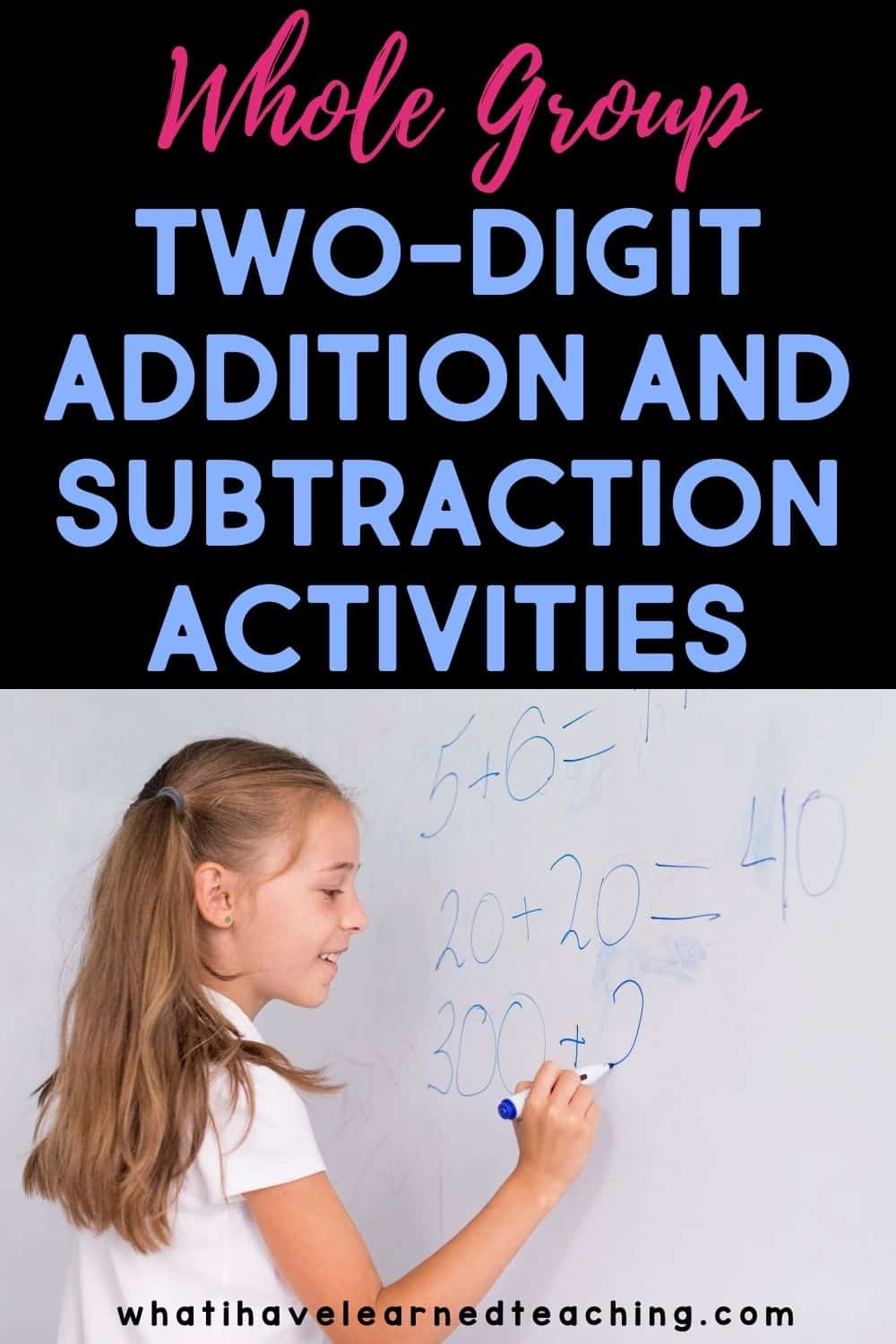# Whole Group Two-Digit Addition & Subtraction Activities

Introducing two-digit addition and subtraction strategies and skills to your learners can be fun and engaging while still meeting national and state math standards. I love using whole group instruction to make sure students are getting a common message about how to use number lines, base ten blocks, place value, and compensation strategies.

These activities meet Common Core State Standard 2.NBT.B.9, (Explain why addition and subtraction strategies work, using place value and the properties of operations) and CCSS 2.NBT.B.5, (Fluently add and subtract within 100 using strategies based on place value, properties of operations, and/or the relationship between addition and subtraction).

Here are some whole group two-digit addition and subtraction activities that meet the standards.

## Number Lines

Since an open number line is so flexible, it lends itself to addition and subtraction regardless of the numbers involved. The concrete action of manipulating numbers on a number line reinforces mathematical thinking. Start by modeling and using a number line with easier problems in whole group instruction. This will make students more comfortable when using it later on with more challenging calculations. Use no-prep two-digit addition and subtraction printables to help your learners use a number line to add and subtract two-digit numbers.

## Flap Books

Teach your whole class how to create two-digit subtraction flap books to practice two-digit subtraction. After whole group instruction, you can also use flap books to differentiate with your learners. Here are some skills included in the books to help your learners solidify their understanding of two-digit subtraction:

• Just tens (Ex: 50 – 20)
• Tens and Ones with a 0 in subtrahend (Ex: 45 – 20)
• Without regrouping (Ex: 67 – 32)
• With regrouping (Ex: 81 – 23)
• Mixed problems

## Base Ten Blocks

Manipulatives can be lifesavers for some students. Drawing manipulatives on paper can save you time and money! Students will always have a pencil and paper, but they will not always have manipulatives to use. No-prep two-digit addition and subtraction printables introduce base ten blocks to illustrate and build two-digit numbers and add and subtract them. Have your students use the resources that allow them to record equations and draw base-10 blocks using symbols like squares, sticks, and dots.

## Two-Digit Number Puzzles

When two-digit addition and subtraction can feel more like a game, your students will get excited to learn! These two-digit number puzzles meet the state and national standards and help your learners visualize how addition and subtraction patterns emerge.

The Decompose Two-Digit Number Puzzles are a four-piece puzzles that help your elementary learners practice two-digit addition and subtraction. Introduce this activity to the whole group to assist in regrouping.

Each puzzle is built with four number relationships. For example:

Practice with the whole group by matching all four pieces or differentiate instruction and concentrate on two pieces at a time.

### What Prior Knowledge Should Students Have?

Before getting started with two-digit addition and subtraction, what should your class know and be able to do?

• automaticity with addition and subtraction facts -memorization isn’t necessary, but speed helps
• ability to find friendly numbers – 10 is a benchmark number, so it’s helpful to quickly know tens
• adding ten to a number – having this foundational skill means that students can effectively see the pattern of adding ten to a number
• place value – students must have a strong understanding of ones and tens. Fluency with place value will also make your student more successful in math.

Although there are many models and strategies for teaching two-digit addition and subtraction, these whole group two-digit addition and subtraction activities that meet the standards prove particularly effective and easy to use with students. By practicing these strategies, math can be more enjoyable for all students.